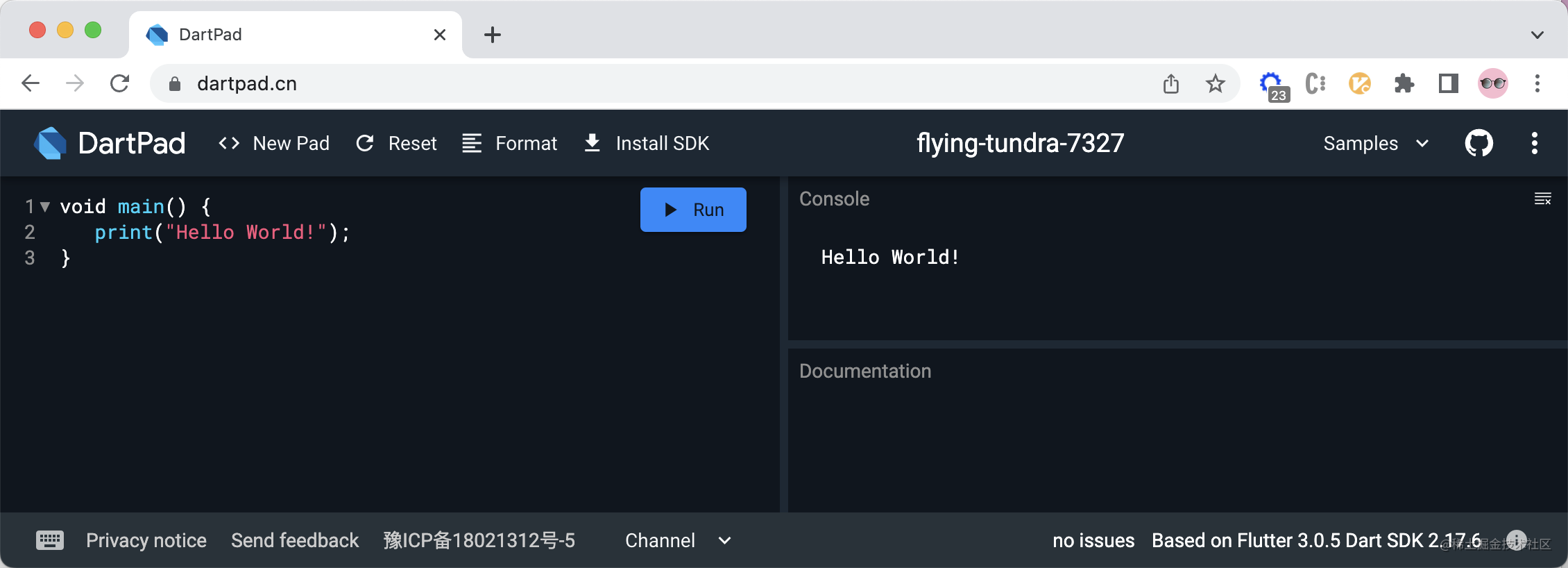# Dart 知识点 - 数据类型

Dart 这个知识点，会写成一个系列的文章。我们以数据类型开始～### Number 数字类型

• Number 数字类型，包含
• int 整型
• double 浮点型

`int``double` 都是 num 的子类。

```bash int a = 1; print(a); // 1

double b = 2.0; print(b); // 2.0

num c = -3; print(c); // -3 ```

### String 字符串类型

`Dart` 支持使用双引号和单引号来创建字符串。使用 `String` 来定义变量，表明该变量是字符串类型。`Dart` 中字符串类型的使用跟 `JavaScript` 中字符串的使用相似：

```bash String s1 = 'Hello '; String s2 = 'World!'; String s3 = s1 + s2; print(s3); // Hello World!```

```bash String s1 = 'I\'m Jimmy.' print(s1); // I'm Jimmy.

String s2 = "第一行文本\n第二行文本"; print(s2); // 第一行文本 // 第二行文本 ```

```bash ''' 第一行文本 第二行文本 ''' // 或者 """ 第一行文本 第二行文本 """```

```bash String s1 = 'my name is Jimmy.'; print('Hello, ' + s1); // Hello, my name is Jimmy. print('Hello, \${ s1 }'); // Hello, my name is Jimmy. print('Hello, \${ s1.substring(10, 1) }!'); // Hello, Jimmy.```

### Boolean 布尔类型

```bash String str = ''; if(str) { print('true'); } else { print('flase'); }```

```bash Error: A value of type 'String' can't be assigned to a variable of type 'bool'. if(str) { ^ Error: Compilation failed.````if(str)` 这里，编译失败，因为在 `dart` 语言里面，变量值必须是 `true` 或者 `false` 才行。改写下上面的代码：`if(str) => if(str.isEmpty)` 即可通过编译。

| | JavaScript (false) | Dart (true) | | --- | --- | --- | | var variable = 0 | variable （false） | variable <= 0 (true) | | var variable = null | variable （false） | variable == null (true) | | var variable = '' | variable （false） | variable.isEmpty (true) | | var variable = NaN | variable （false） | variable.isNaN (true) | | var variable = true | variable （true） | variable (true) |

```bool flag = true // 或者 bool flag = false```

### list 数组类型

```bash List arr = ['Hello ', 'World!']; // 数组的长度 print(arr.length); // 2 // 数组的取值 print(arr); // Hello // 数组的赋值 arr = 'Jimmy. '; print(arr.join('')); // Hello Jimmy. // 扩展运算符 var list = ["I\'m ", 'come ', 'from ', 'China.']; print([...arr, ...?list].join('')); // Hello Jimmy. I'm come from China.```

`...?` 为空感知扩展操作符，如果扩展操作符右边的变量值可能是 `null`， 我们可以使用 `...?` 来避免产生异常

### Set 集合类型

• 无序性：每个元素的地位都是相同的，元素之间无序。
• 互异性：每个元素只能出现一次。
• 确定性：给定一个集合，任何一个元素，该元素`或者属于或者不属于`该集合，二者必居其一，不允许模棱两可的情况出现。

```bash // 定义集合 Set set = new Set(); // 集合的大小 print(set.length); // 0 // 添加元素 set.add('Hello '); set.add('World!'); print(set); // {Hello , World!} // 判断集合中是否存在指定元素 print(set.contains('Jimmy')); // false // 一次添加多个列表元素 set.addAll(["I\'m ", 'come ', 'from ', 'China.']); print(set); // {Hello , World!, I'm , come , from , China.} // 移除指定的元素 set.remove('World!'); print(set); // {Hello , I'm , come , from , China.}```

### Map 对象类型

`Map` 理解成对象，是因为这跟 `JavaScript` 中的对象很类似。

```bash // 定义对象 Map map = new Map(); // 对象的大小 print(map.length); // 0 // 添加对象属性 map['name'] = 'Jimmy'; map['country'] = 'China'; print(map); // {name: Jimmy, country: China} // 读取属性值 print(map['name']); // Jimmy // 读取对象的 keys 值 print(map.keys); // (name, country) // 读取对对象的 values 值 print(map.values); // (Jimmy, China)```

### Runes 和 Symbols

`Dart` 中，runes 公开了字符串的 Unicode 码位。Symbol 表示 Dart 中声明的操作符或者标识符。

### 补充

`var``Dart` 语言使用的关键字，是 `variable` 的简写，表明这是一个泛型调用（generic invocations）

```bash var name = 'Jimmy'; // 泛型调用，表明变量类型不知道是啥 String name = 'Jimmy'; // 类型注释，表明我一定是 String 类型```